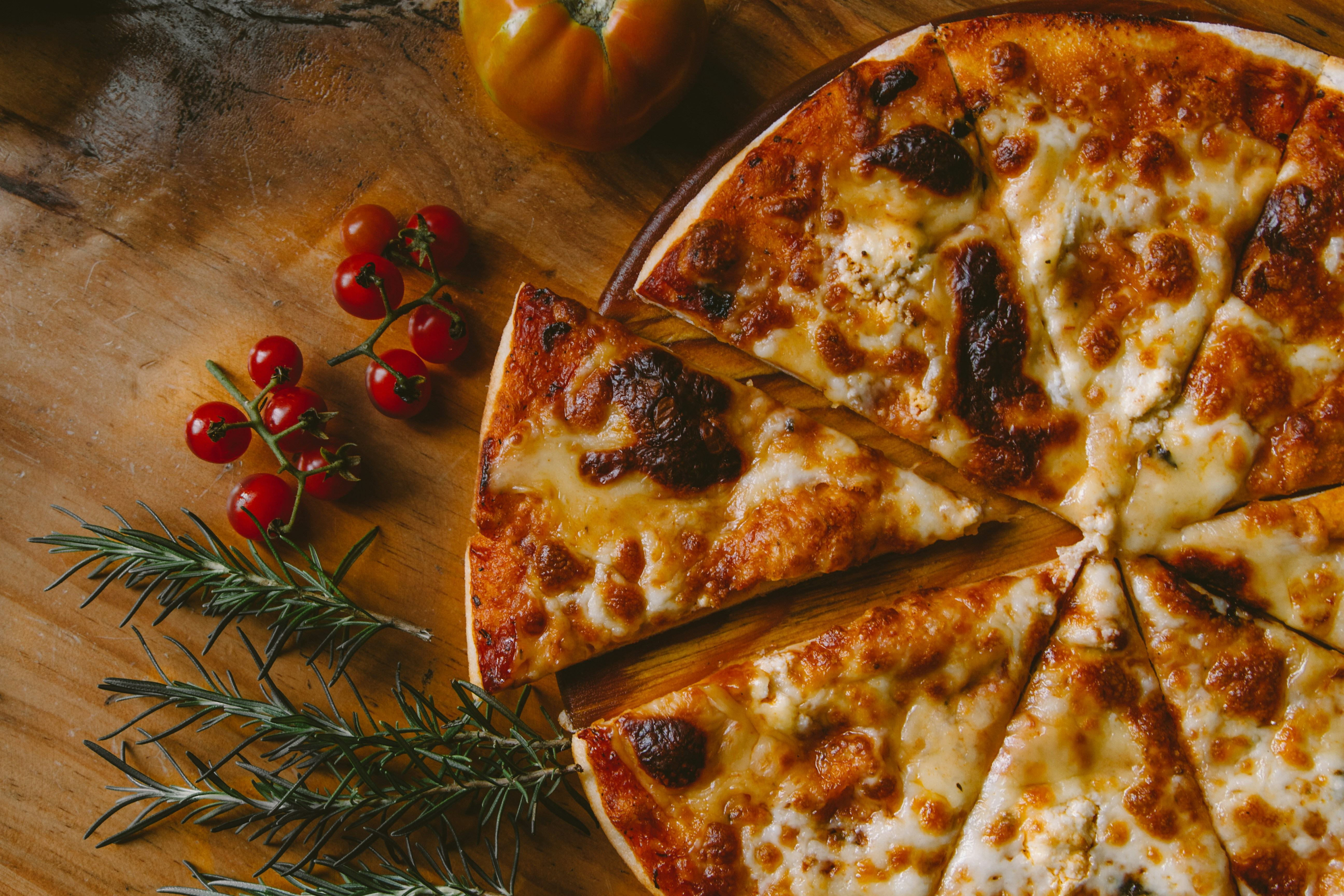# My Mistake On Converting Slice To Slice Of Ptr In Golang

Apr 22, 2021  ·  ~1min read
Notes #golang #pointer #sliceI once made a function to convert a slice to slice of ptr and made a mistake.

## My Function

``````func Slice2SliceOfPtr(slice []int) []*int {
n := len(slice)
r := make([]*int, n, n)

for i, s := range slice {
r[i] = &s
}

return r
}
``````

It seems normal to me until I realize something was wrong.

## My Main Func

``````func main() {
x := []int{1, 2, 3}
y := Slice2SliceOfPtr(x)

fmt.Println(x)

for _, yy := range y {
fmt.Printf("%d ", *yy)
}
}
``````

## Result

``````\$ go run main.go
[1 2 3]
3 3 3
``````

Why only the last element copied to the result?

Because the `&s` I used is mutated every iteration.

``````for i, s := range slice {
r[i] = &s
}
``````

`s` never created as a new variable on every iteration, but its value is mutated inside the for loop

So I changed the iteration like this:

## Better One

``````for i := range slice {
r[i] = &slice[i]
}
``````
Thank you for reading!